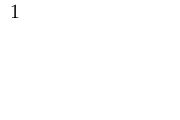×

Search anything:

# Bell Numbers: Number of partitions of a set

#### Algorithms List of Mathematical AlgorithmsGet this book -> Problems on Array: For Interviews and Competitive Programming

Reading time: 15 minutes | Coding time: 4 minutes

The Bell numbers are a sequence of numbers that describe the number of ways a set with N elements can be partitioned into disjoint, non-empty subsets.

The first few Bell numbers are:


1, 1, 2, 5, 15, 52, 203, 877, 4140, 21147, 115975, 678570, 4213597, ...


Where Bn represesnts the number of disjoint sets that the array can be partitioned into.

Note : B0 is 1 because there is exactly one partition of the empty set.

### Algorithm

The Bell Number for a set of particular size (Bn) can be calculated in quite many interesting ways, We will be exploring 3 particular methods:

• Dobinski's Formula
• Sum of Sterling's number of second kind
• The Peirce Triangle Method

### Calculating Bell Numbers using Dobinski's Formula

Nth Bell number using Dobinski's Formula is given by:

$$B(n)=\frac{1}{e}\sum_{j=0}^{\infty}\frac{j^n}{j!}.$$

The time complexity is exponential that is $N^2$.

Pseudocode:

N = [The n in Bn];
sum = 0;
iterations =[ A very large number (>=10000)  ];
for (i = 0; i < iterations ; i++)
sum = sum + ((pow(i,N)/(i!))
print (sum/e)


### Calculating Bell Numbers using Summation Of Stirling's Second kind

The Bell number $B(n)$ is defined as $\sum_{k=1}^n S(n,k)$ where $S(n,k)={n\brace k}$ is a Stirling number of the second kind.

Stirling number is given as:

$${n\brace k} = \frac{1}{k!}\sum_{i=0}^{k}{k \choose i}i^n(-1)^{k-i}$$

and for n == k or k = 1, the Stirling number is 1.

Pseudocode:

N = [The n in Bn];
sum = 0;

B(N) = S(N,k) for k = 1 to N

function S(n,k){
if n == 0 or k == 0 or k > n
return 0
else {
if k == 1 or n==k
return 1
}
else
return k*S(n-1, k) + S(n-1, k-1)
}


### Calculating Bell Numbers using Peirce's Triangle

This is interestingly the best approach available with a performance better than both the above candidates in cases of N<30. Otherwise, the Dynamic approach is the way to go (see the complexities invloved below)

• Start with the number one. Put this on a row by itself. (x0,1 = 1)
• Start a new row with the rightmost element from the previous row as the leftmost number.
• Determine the numbers not on the left column by taking the sum of the number to the left and the number above the number to the left, that is, the number diagonally up and left of the number we are calculating
• Repeat step three until there is a new row with one more number than the previous row
• The number on the left hand side of a given row is the Bell number for that row.

One such example for better clarification:### Complexity

• Using Dobinski's Formula: Θ(N^2)
• Using Summation Of Sterling's Second kind: Θ(N^2)
• Using Peirce's Triangle: Θ(N^3)

### Implementations

Implementations of the various techniques of computing Bell numbers in Python:

• Python: Dobinski
• Python: Stirling
• Python: Perice's Triangle

### Dobinski's


# Finding Bell Number using Dobinski's Formula
import math
def BellNumbers(N):
ITERATIONS = 10000
ANSWER = (1/math.e) * sum([(k**N)/(math.factorial(k)) for k in range(iterations)])
# Utility code to test this BellNumbers function:
main_set = list(map(int,input().split()))
print ("There are {} ways to split the set : {} into disjoint sets".format(BellNumbers(len(main_set)), main_set))


### Stirling's


# Finding Bell Number using Summation of Sterling's Numbers
def Sterling(N, k):
if n == 0 or k == 0 or k > n:
return 0
if k == 1 or n == k:
return 1
else :
return k*Sterling(N-1, k) + Sterling(N-1, k-1)
def BellNumbers(N):
PARTITIONS = 1
for i in range(N):
PARTITIONS += Sterling(N,i)
return PARTITIONS
# Utility code to test this BellNUmbers function:
main_set = list(map(int,input().split()))
print ("There are {} ways to split the set : {} into disjoint sets".format(BellNumbers(len(main_set)), main_set))


### Perice's


# Finding Bell Number using Peirce's Triangle
def ShowMatrix(matrix):
for i in matrix:
print(*i)
def BellNumbers(N, show=True):
matrix = [[0 for i in range(N+1)] for j in range(N+1)]
matrix = 1
for i in range(1, N+1):
matrix[i] = matrix[i-1][i-1]
for j in range(1, i+1):
matrix[i][j] = matrix[i-1][j-1] + matrix[i][j-1]
if show == True:
print("\n")
for k in matrix:
print (*k)
return (matrix[N])
# Utility code to test this BellNUmbers function:
main_set = list(map(int,input().split()))
print ("There are {} ways to split the set : {} into disjoint sets".format(BellNumbers(len(main_set), show=False), main_set))


### Applications

Applications of Bell numbers are:

• Calculate the number of partition of a set of N numbers

• To find the number of different multiplicative partitions of a square free number N

Fun facts about the bell numbers are that it can also determine the number of rhyming schemes in a poem of n lines!

Bn = Number of rhyme schemes in a poem of n lines